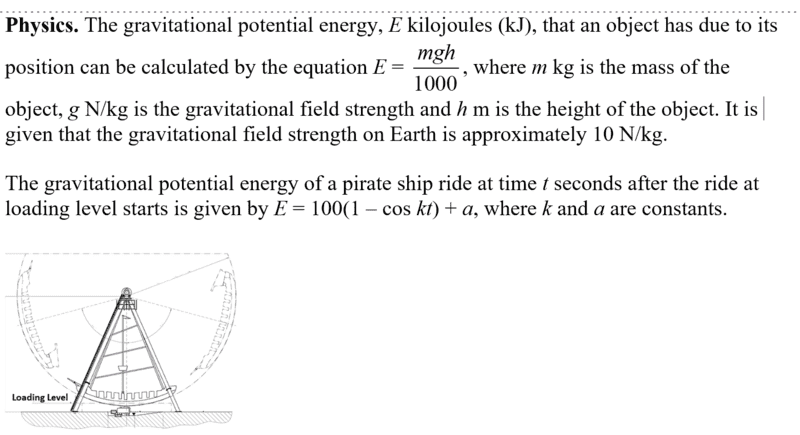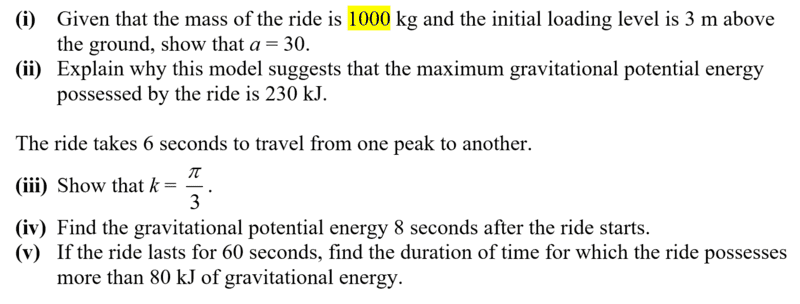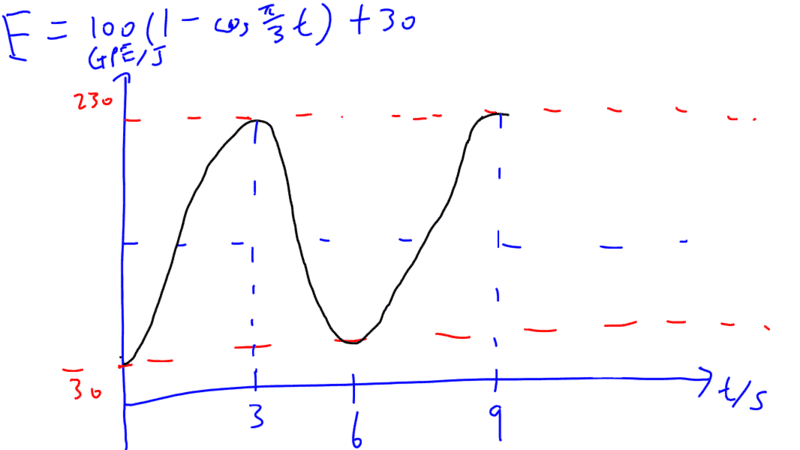# Equation to represent the GPE of a pirate ship ride?

## Homework StatementI came across the question above - and one of my peers told me that the equation E = 100(1-coskt) + a in the question is actually inaccurate in that it does not represent the motion of the pirate ship ride properly, because the equation isn't simple harmonic - the negative example to prove this was that when t = 3, kt = π radians (since k = π/3). I'm not very strong in this topic so I was unable to debate too much with him, so I wanted to get some insights from the experts here.

## Homework Equations

He claims that the equation to represent the GPE of the pirate ship should be E = 100[1-cos (θm sin ωt)] + 30, so that the value of θ varies from 0 to 2. This would make the equation simple harmonic.

## The Attempt at a SolutionI drew the graph above, and I still don't really see any problem with the GPE equation, actually. Could someone please enlighten me on this one?

Thank you so much in advance for any help!

#### Attachments

Chandra Prayaga
I don't see anything wrong with your equation for E. It satisfies every condition laid out in the problem. So, can you now answer the questions (iii), (iv) and (v)?

haruspex